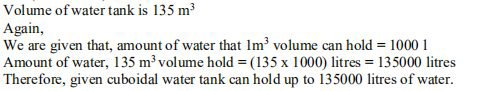# NCERT Solutions Class 9 Mathematics Solutions for Surface Areas and Volumes - Exercise 13.5 in Chapter 13 - Surface Areas and Volumes

A cuboidal water tank is 6 m long, 5 m wide and 4.5 m deep. How many liters of water can

Does it hold? \left(1 \mathrm{m}^{3}=1000 \mathrm{l}\right)

Dimensions of a cuboidal water tank are: l = 6 m and b = 5 m and h = 4.5 m

Formula to find the volume of the tank , V = l x b x h

Put the values, we get

V = (6 x 5 x 4.5) = 135Related Questions

Lido

Courses

Teachers

Book a Demo with us

Syllabus

Maths
CBSE
Maths
ICSE
Science
CBSE

Science
ICSE
English
CBSE
English
ICSE
Coding

Terms & Policies

Selina Question Bank

Maths
Physics
Biology

Allied Question Bank

Chemistry
Connect with us on social media!